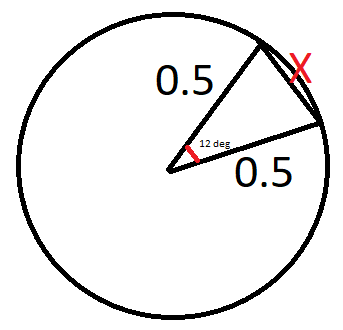# Help me out on a math problem? lol. Trigonometry related

Basically, I’m trying to generate a circle using frames, and I need some help with the maths to get the frames to be the correct size.

Long story short,

How would I find the length of side x in this diagram?The trigonometry I know deals with right triangles, which this is not. Thanks in advance!!

Search up the cosine law. I don’t know how to do math formatting on here, but you can search up the formula on google. X should be about 0.1

With isosceles triangles you can split them in two so they are symmetrical.
This means that you are left knowing 12/2 = 6 degrees at the smallest angle and one side remains as 0.5
Now that we have a right angled triangle can use SohCahToa to find our formula: sin(6) = x/0.5
Now we can re-arrange the equation to x = 0.5*sin(6) to get a grand total of ~0.1

Thanks WeeklyShoe for correcting me on the rearrangement (it is correct now)

math.random(-y, y)

the rearranged formula should be x = 0.5sin(6), but that’s only half of X. so X should be sin(6) which is about 0.1

4 Likes